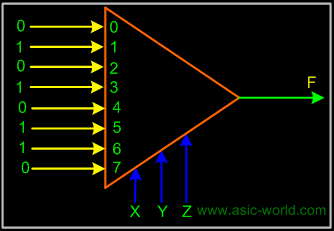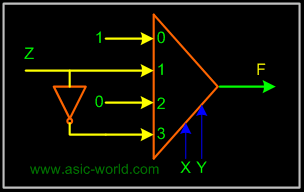# Trung tâm đào tạo thiết kế vi mạch Semicon

• Đăng ký
 Name: * Tên đăng nhập: * Email: * Mật khẩu: * Verify Password: * Fields marked with an asterisk (*) are required.## Digital Combinational Logic Part-VIIBoolean Function Implementation

Earlier we had seen that it is possible to implement Boolean functions using decoders. In the same way it is also possible to implement Boolean functions using muxers and de-muxers.

Implementing Functions Multiplexers
Any n-variable logic function can be implemented using a smaller 2n-1-to-1 multiplexer and a single inverter (e.g 4-to-1 mux to implement 3 variable functions) as follows.
Express function in canonical sum-of-minterms form. Choose n-1 variables as inputs to mux select lines. Construct the truth table for the function, but grouping inputs by selection line values (i.e select lines as most significant inputs).

Determine multiplexer input line i values by comparing the remaining input variable and the function F for the corresponding selection lines value i.
We have four possible mux input line i values:

• Connect to 0 if the function is 0 for both values of remaining variable.
• Connect to 1 if the function is 1 for both values of remaining variable.
• Connect to remaining variable if function is equal to the remaining variable.
• Connect to the inverted remaining variable if the function is equal to the remaining variable inverted.

Example: 3-variable Function Using 8-to-1 mux
Implement the function F(X,Y,Z) = S(1,3,5,6) using an 8-to-1 mux. Connect the input variables X, Y, Z to mux select lines. Mux data input lines 1, 3, 5, 6 that correspond to the function minterms are connected to 1. The remaining mux data input lines 0, 2, 4, 7 are connected to 0.Example: 3-variable Function Using 4-to-1 mux

 Implement the function F(X,Y,Z) = S(0,1,3,6) using a single 4-to-1 mux and an inverter. We choose the two most significant inputs X, Y as mux select lines.

Truth Table

 Select i X Y Z F Mux Input i 0 0 0 0 1 1 0 0 0 1 1 1 1 0 1 0 0 Z 1 0 1 1 1 Z 2 1 0 0 0 0 2 1 0 1 0 0 3 1 1 0 1 Z' 3 1 1 1 0 Z'
• Circuit
•• We determine multiplexer input line i values by comparing the remaining input variable Z and the function F for the corresponding selection lines value iwhen XY=00 the function F is 1 (for both Z=0, Z=1) thus mux input0 = 1
• when XY=01 the function F is Z thus mux input1 = Z
• when XY=10 the function F is 0 (for both Z=0, Z=1) thus mux input2 = 0
• when XY=11 the function F is Z' thus mux input3 = Z'
Example: 2 to 4 Decoder using Demux
•Mux-Demux Application Example
This enables sharing a single communication line among a number of devices. At any time, only one source and one destination can use the communication line.
•• Bạn Có Đam Mê Với Vi Mạch hay Nhúng      -     Bạn Muốn Trau Dồi Thêm Kĩ Năng

# Hãy Để Chúng Tôi Hỗ Trợ Cho Bạn. SEMICONLần cập nhật cuối ( Thứ ba, 29 Tháng 3 2022 00:12 )